# Normal Probability Plots for Dummies

Recently, while working on a project, I had to verify that a set of data points was normally distributed. An easy, qualitative way to do this is to create a normal probability plot. The only problem was that I completely forgot how to do this, and all the explanations that I could find online sucked! So, here's a dead simple primer on normal probability plots, for boneheads like me with only basic statistics knowledge.

Let's say we have some dataset. In my case, it's the value of a pixel in an image over time. We can sort the elements of the dataset in ascending order, which allows us to assign each element a rank. In addition, let's also compute the z-score of each element.

Value Rank z-score
2062 1 -4.76
2069 2 -4.72
2122 3 -4.43
2170 4 -4.16
2216 5 -4.01
...

Now, here's the trick. We can use some math to determine the expected z-score of the nth element in a normally distributed dataset with the same size as ours. For example, we might say that the expected z-score of the 50th element in a sample of 100 normally distributed values is 0.

Using this, we can create a plot of our points where the x-axis is the expected z-score, and the y-axis is the actual z-score. If the distribution is really normal, the resulting points will fall on a straight line. Any deviations may tell you information about the true distribution, such as its skewness.

So how do we actually compute that expected z-score I just mentioned? Well, we can start with the normal CDF, which we'll write as $\Phi(x)$. This function tells us the probability of a normally distributed random value being less than $x$.

Notice that the CDF does the exact opposite of what we want. If we're considering the nth value of a set, we know the probability of a normal random variable being smaller than the point we're looking at, and want to know the z-score. Therefore, we should use the quantile function, which is the inverse of the CDF. For this reason, we'll write it as $\Phi^{-1}(x)$.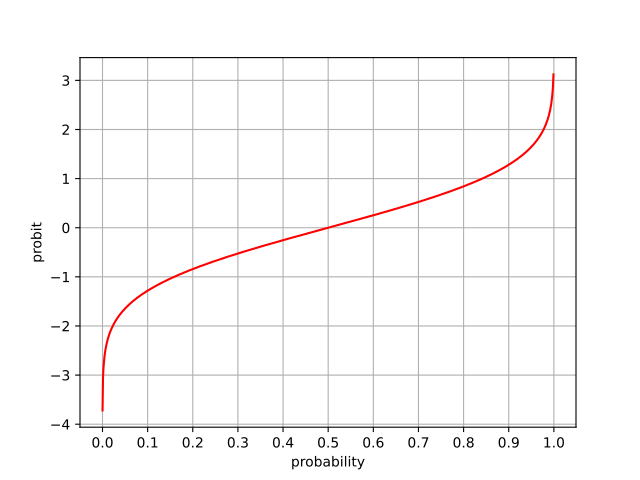The quantile function of a standard normal distribution, also known as the probit function.

Now let's write a formula that converts rank $n$ and dataset size $N$ to z-score $z$. We need to be careful since $\Phi^{-1}(x)$ diverges at $0$ and $1$. The following formula is used in many statistics packages:

$z = \Phi^{-1}\left(\frac{n - \frac12}{N}\right)$

Using this, we can assign our datapoints an expected z-score...

Value Rank z-score Expected z-score
2062 1 -4.76 -2.97
2069 2 -4.72 -2.62
2122 3 -4.43 -2.44
2170 4 -4.16 -2.32
2216 5 -4.01 -2.23
...

...and draw our normal probability plot: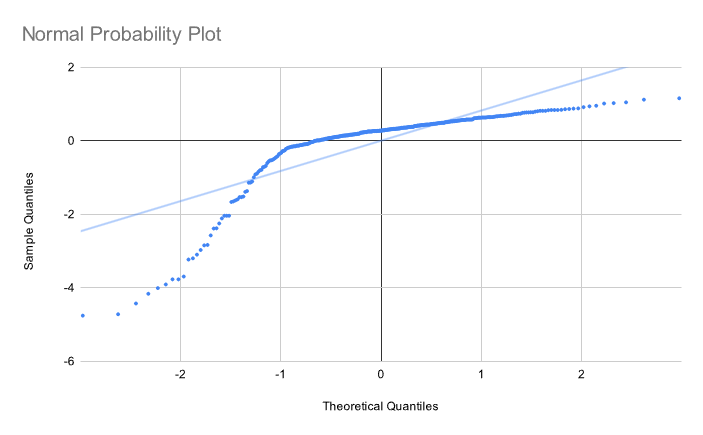Oof. Not very normal at all. This is corroborated by the histogram: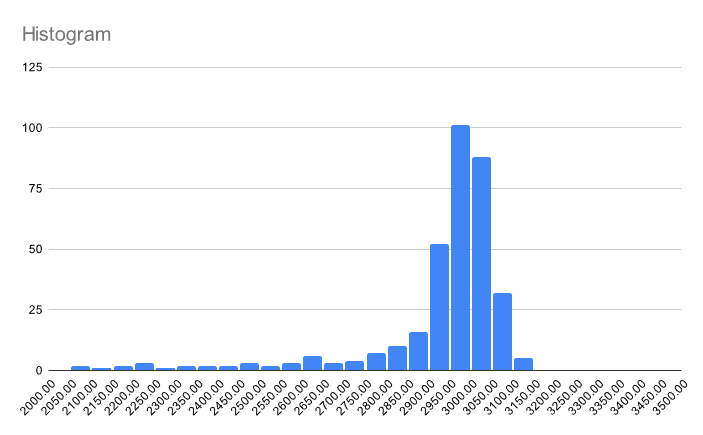Let's try again, this time on a different set that is actually normally distributed. The normal probability plot: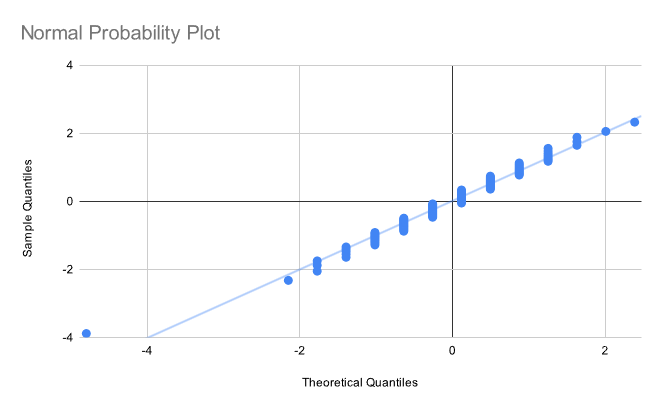and the histogram: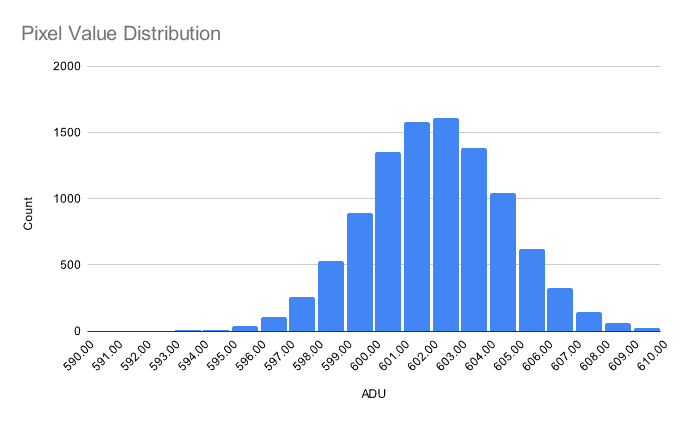Nice! The probability plot looks a little weird due to quantization of the x-values, but this doesn't affect its functionality.

To learn more, I recommend checking out the section on the Normal Probability Plot from NIST's engineering statistics handbook.